Thermodynamics and Propulsion

# 7.4 Connection between the Statistical Definition of Entropy and Randomness

We need now to examine the behavior of the statistical definition of entropy as regards randomness. Because a uniform probability distribution reflects the largest randomness, a system with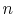allowed states will have the greatest entropy when each state is equally likely. In this situation, the probabilities become(7..15)

whereis the total number of microstates. The entropy is thus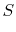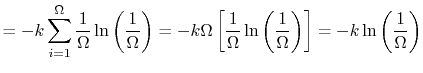(7..16)

Equation (7.16) states that the larger the number of possible states the larger the entropy. The behavior of the entropy stated in Equation (7.16) can be summarized as follows:
1.is maximum whenis maximum, which means many permitted quantum states, hence much randomness,
2.is minimum whenis minimum. In particular, for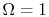, there is no randomness and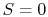.
These trends are in accord with our qualitative ideas concerning randomness. Equation (7.16) is carved on Boltzmann's tombstone (he died in 1906 in Vienna).

We can also examine the additive property of entropy with respect to probabilities. If we have two systems,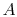and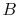, which are viewed as a combined system,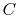, the quantum states for the combined system are the combinations of the quantum states fromand. The quantum state whereis in its state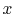andis in its state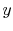would have a probabilitybecause the two probabilities are independent. The number of probabilities for the combined system,, is thus defined by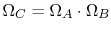. The entropy of the combined system is(7..17)

Equation (7.16) is sometimes taken as the basic definition of entropy, but it should be remembered that it is only appropriate when each quantum state is equally likely. Equation (7.12) is more general and applies equally for equilibrium and non-equilibrium situations.

A simple numerical example shows trends in entropy changes and randomness for a system which can exist in three states. Consider the five probability distributions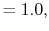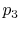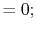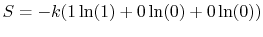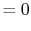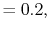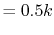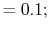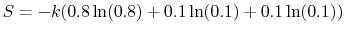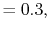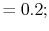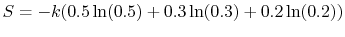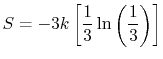The first distribution has no randomness. For the second, we know that state 3 is never found. Distributions (iii) and (iv) have progressively greater uncertainty about the distribution of states and thus higher randomness. Distribution (v) has the greatest randomness and uncertainty and also the largest entropy.

UnifiedTP